# Selina Solutions Concise Mathematics Class 6 Chapter 12: Proportion Exercise 12(A)

Selina Solutions Concise Mathematics Class 6 Chapter 12 Proportion Exercise 12(A) explains the basic knowledge of Proportion in ratios. Referring to solved examples before each exercise helps students in clearing their doubts instantly. The solutions are designed by experts in a stepwise manner, as per the latest ICSE exam guidelines. This also helps students to rectify their weaknesses and work on them for a better academic performance. Students can download Selina Solutions Concise Mathematics Class 6 Chapter 12 Exercise 12(A) PDF free, from the links provided below

## Selina Solutions Concise Mathematics Class 6 Chapter 12 Proportion Exercise 12(A) Download PDF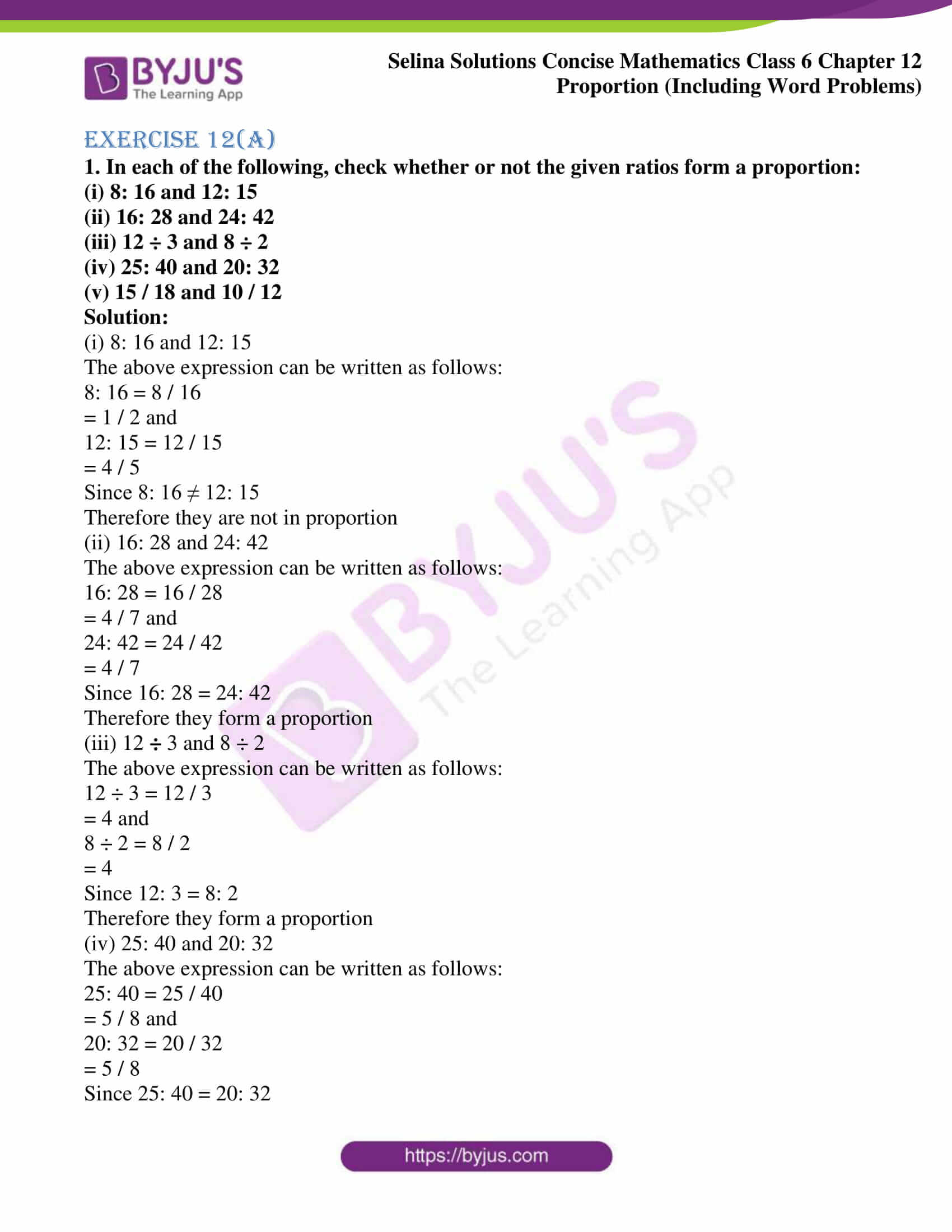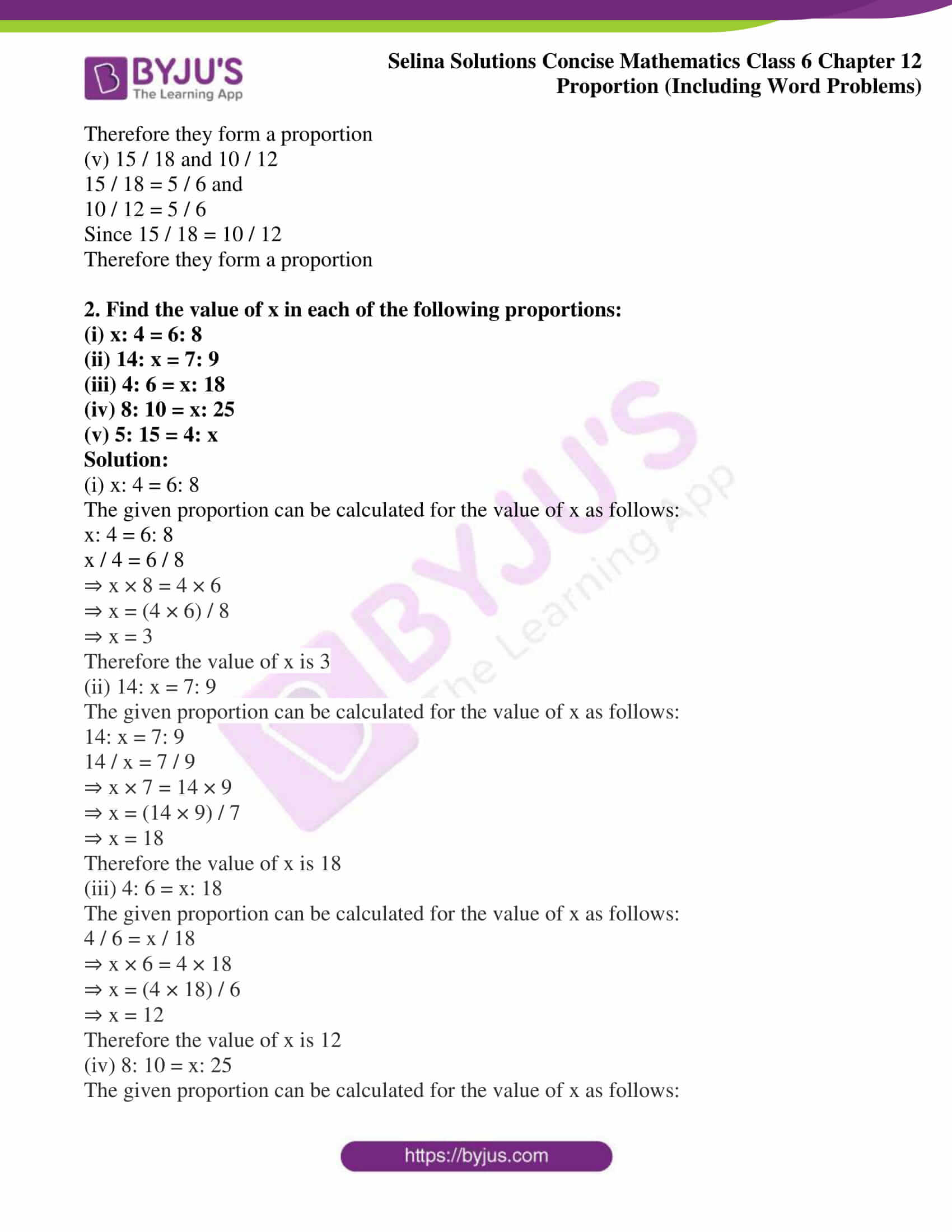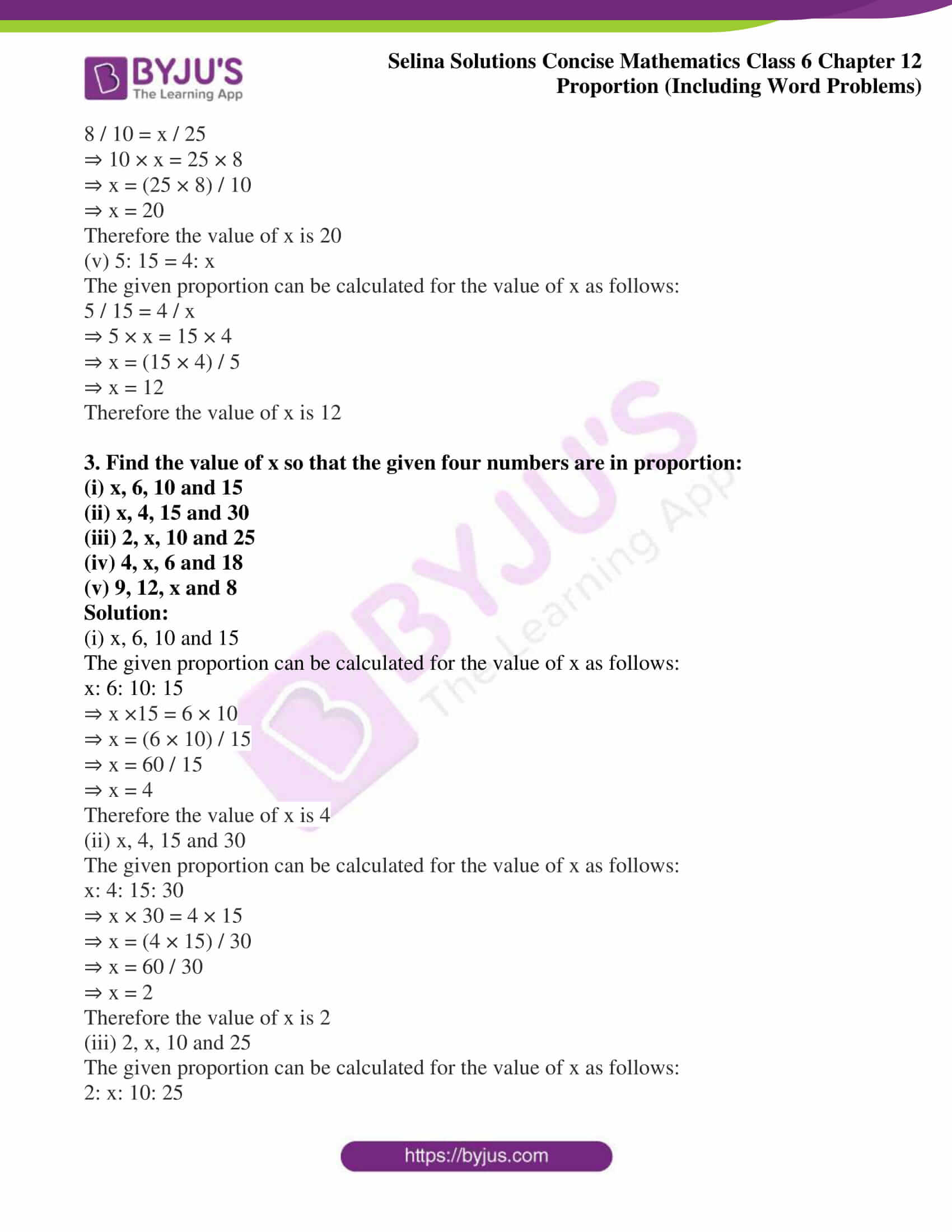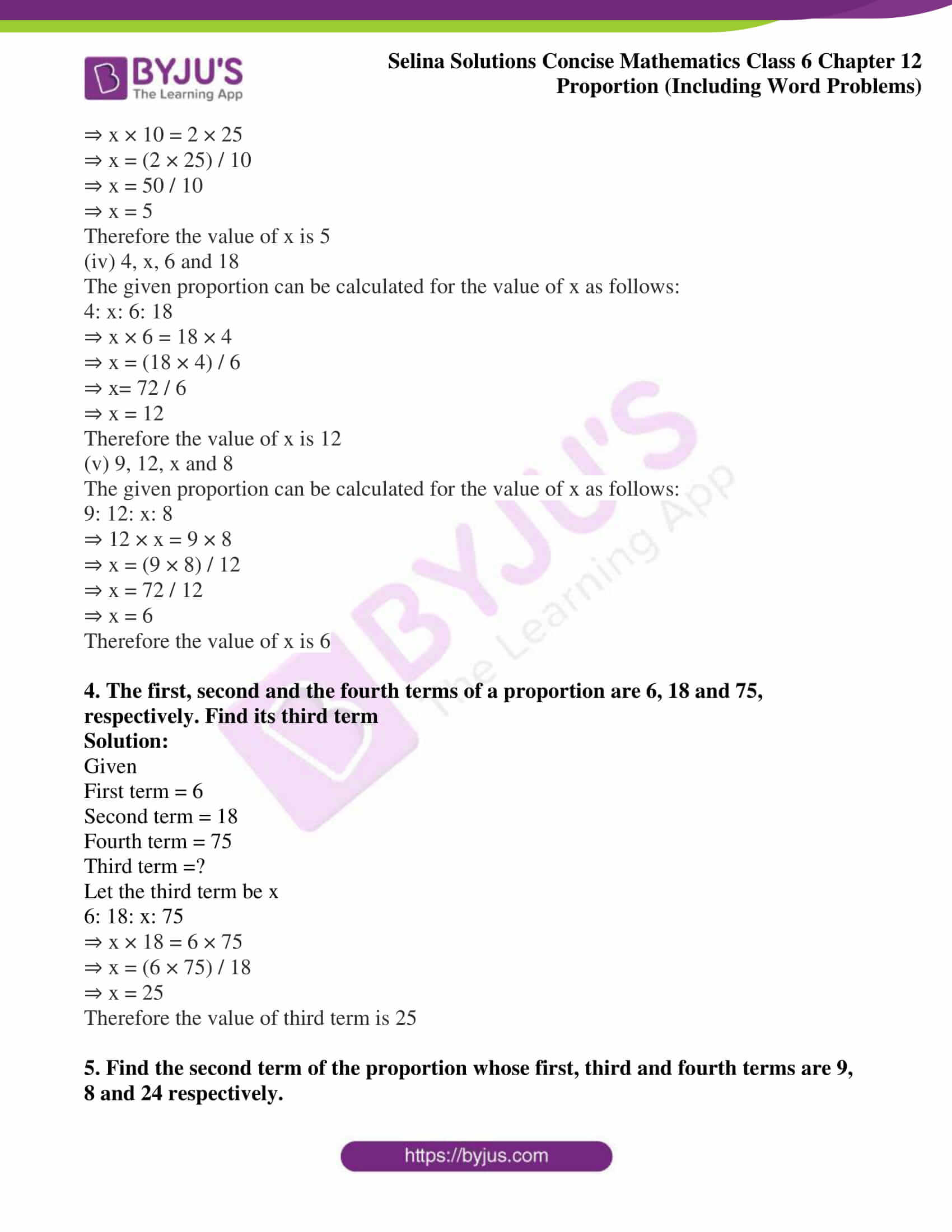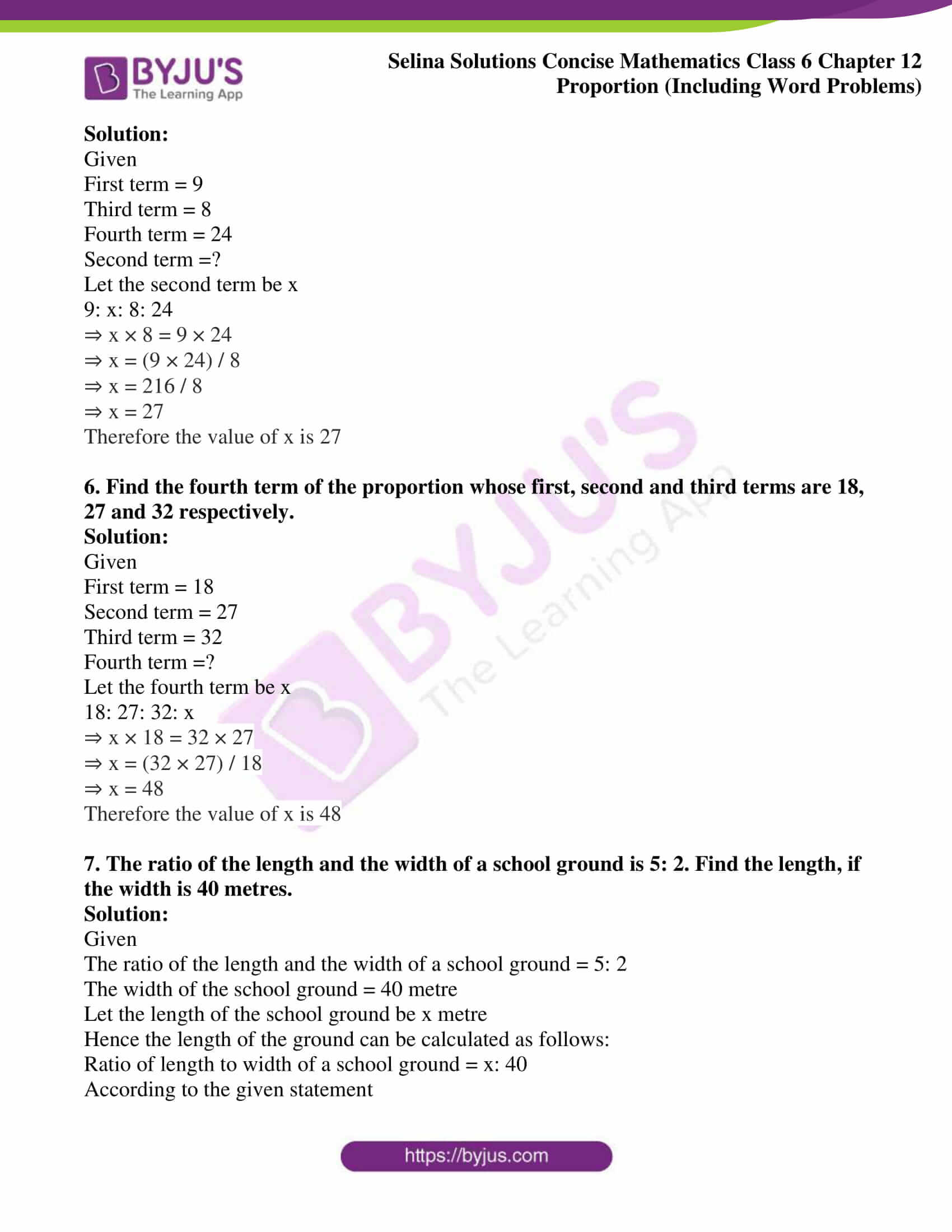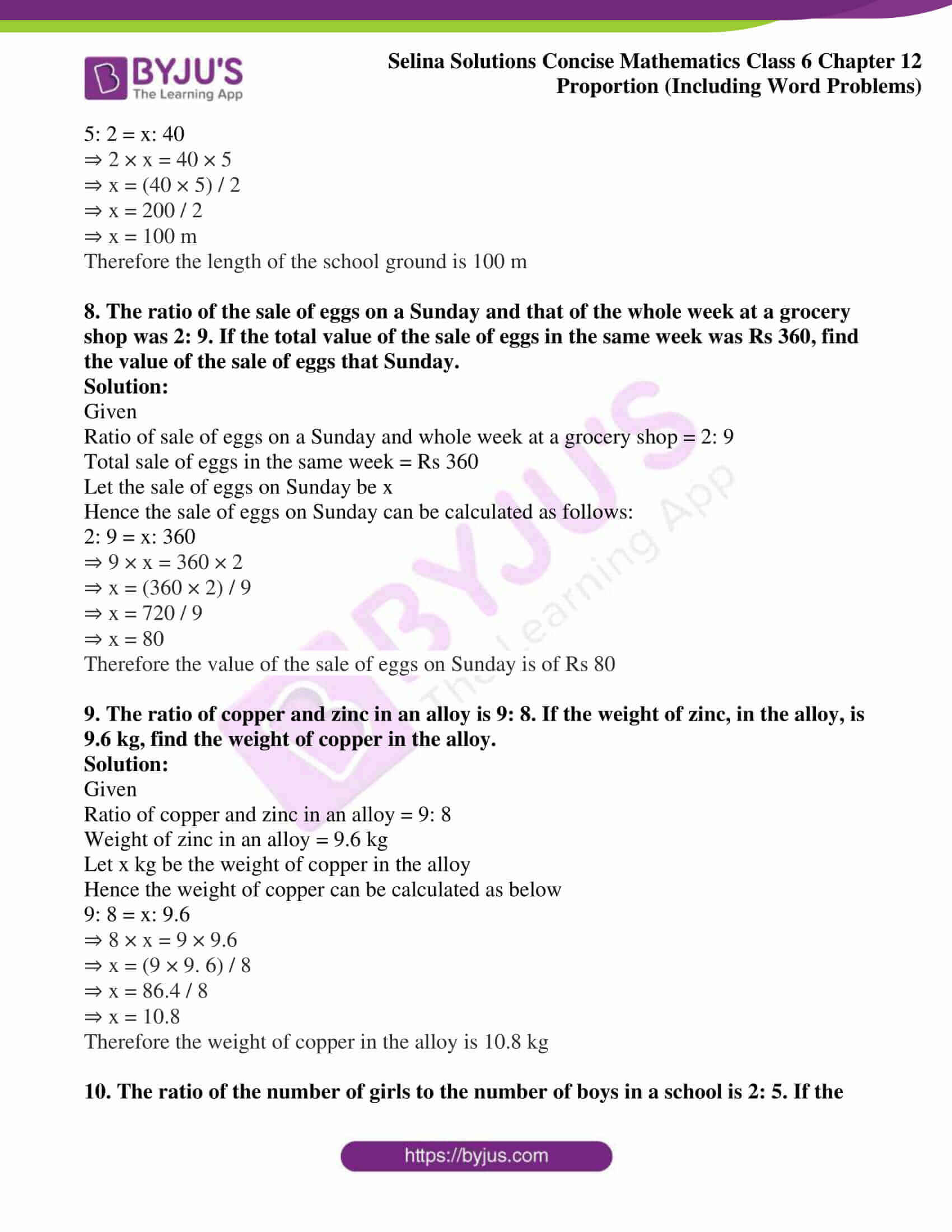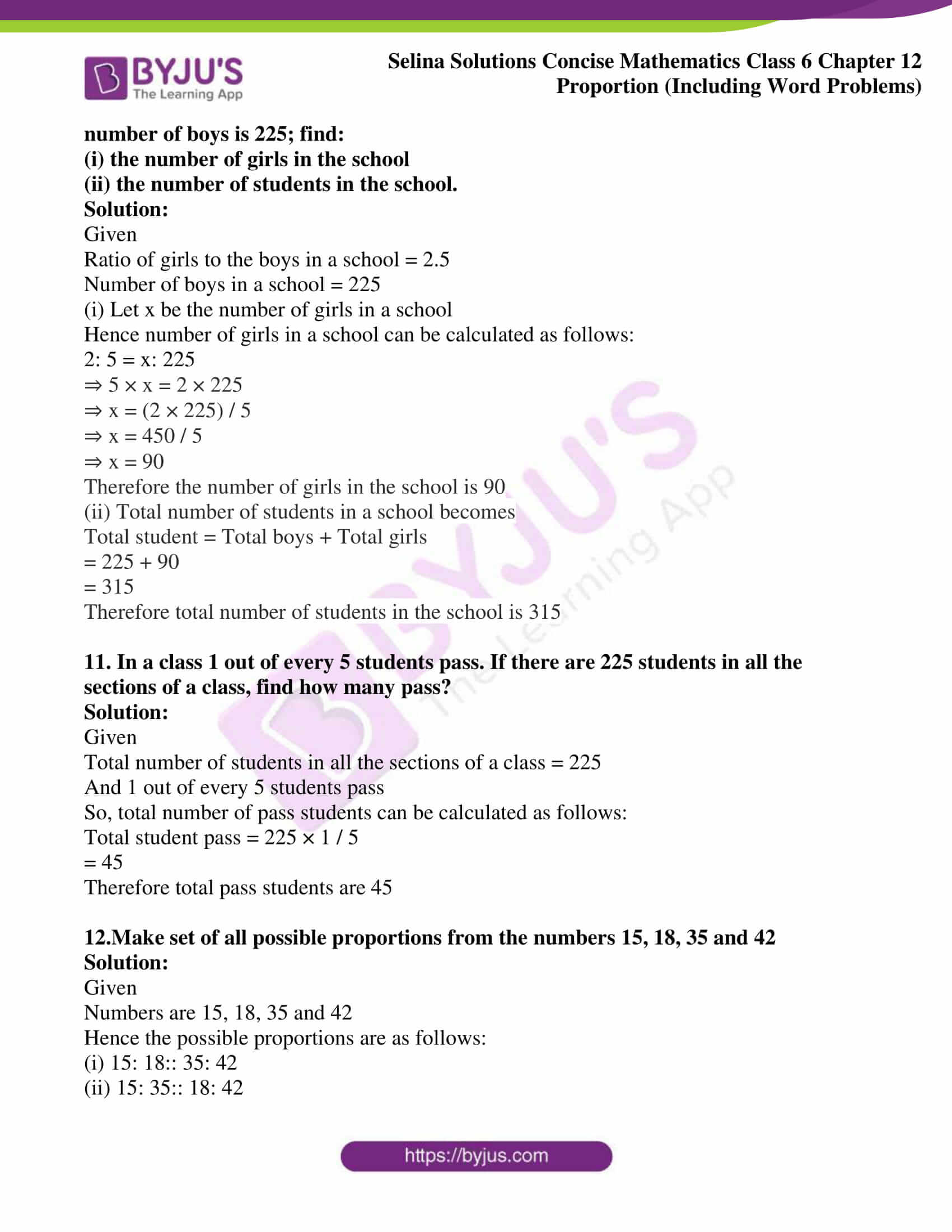### Access other exercises of Selina Solutions Concise Mathematics Class 6 Chapter 12 Proportion

Exercise 12(B) Solutions

Exercise 12(C) Solutions

### Access Selina Solutions Concise Mathematics Class 6 Chapter 12 Proportion Exercise 12(A)

Exercise 12(A)

1. In each of the following, check whether or not the given ratios form a proportion:

(i) 8: 16 and 12: 15

(ii) 16: 28 and 24: 42

(iii) 12 ÷ 3 and 8 ÷ 2

(iv) 25: 40 and 20: 32

(v) 15 / 18 and 10 / 12

Solution:

(i) 8: 16 and 12: 15

The above expression can be written as follows:

8: 16 = 8 / 16

= 1 / 2 and

12: 15 = 12 / 15

= 4 / 5

Since 8: 16 ≠ 12: 15

Therefore they are not in proportion

(ii) 16: 28 and 24: 42

The above expression can be written as follows:

16: 28 = 16 / 28

= 4 / 7 and

24: 42 = 24 / 42

= 4 / 7

Since 16: 28 = 24: 42

Therefore they form a proportion

(iii) 12 ÷ 3 and 8 ÷ 2

The above expression can be written as follows:

12 ÷ 3 = 12 / 3

= 4 and

8 ÷ 2 = 8 / 2

= 4

Since 12: 3 = 8: 2

Therefore they form a proportion

(iv) 25: 40 and 20: 32

The above expression can be written as follows:

25: 40 = 25 / 40

= 5 / 8 and

20: 32 = 20 / 32

= 5 / 8

Since 25: 40 = 20: 32

Therefore they form a proportion

(v) 15 / 18 and 10 / 12

15 / 18 = 5 / 6 and

10 / 12 = 5 / 6

Since 15 / 18 = 10 / 12

Therefore they form a proportion

2. Find the value of x in each of the following proportions:

(i) x: 4 = 6: 8

(ii) 14: x = 7: 9

(iii) 4: 6 = x: 18

(iv) 8: 10 = x: 25

(v) 5: 15 = 4: x

Solution:

(i) x: 4 = 6: 8

The given proportion can be calculated for the value of x as follows:

x: 4 = 6: 8

x / 4 = 6 / 8

⇒ x × 8 = 4 × 6

⇒ x = (4 × 6) / 8

⇒ x = 3

Therefore the value of x is 3

(ii) 14: x = 7: 9

The given proportion can be calculated for the value of x as follows:

14: x = 7: 9

14 / x = 7 / 9

⇒ x × 7 = 14 × 9

⇒ x = (14 × 9) / 7

⇒ x = 18

Therefore the value of x is 18

(iii) 4: 6 = x: 18

The given proportion can be calculated for the value of x as follows:

4 / 6 = x / 18

⇒ x × 6 = 4 × 18

⇒ x = (4 × 18) / 6

⇒ x = 12

Therefore the value of x is 12

(iv) 8: 10 = x: 25

The given proportion can be calculated for the value of x as follows:

8 / 10 = x / 25

⇒ 10 × x = 25 × 8

⇒ x = (25 × 8) / 10

⇒ x = 20

Therefore the value of x is 20

(v) 5: 15 = 4: x

The given proportion can be calculated for the value of x as follows:

5 / 15 = 4 / x

⇒ 5 × x = 15 × 4

⇒ x = (15 × 4) / 5

⇒ x = 12

Therefore the value of x is 12

3. Find the value of x so that the given four numbers are in proportion:

(i) x, 6, 10 and 15

(ii) x, 4, 15 and 30

(iii) 2, x, 10 and 25

(iv) 4, x, 6 and 18

(v) 9, 12, x and 8

Solution:

(i) x, 6, 10 and 15

The given proportion can be calculated for the value of x as follows:

x: 6: 10: 15

⇒ x ×15 = 6 × 10

⇒ x = (6 × 10) / 15

⇒ x = 60 / 15

⇒ x = 4

Therefore the value of x is 4

(ii) x, 4, 15 and 30

The given proportion can be calculated for the value of x as follows:

x: 4: 15: 30

⇒ x × 30 = 4 × 15

⇒ x = (4 × 15) / 30

⇒ x = 60 / 30

⇒ x = 2

Therefore the value of x is 2

(iii) 2, x, 10 and 25

The given proportion can be calculated for the value of x as follows:

2: x: 10: 25

⇒ x × 10 = 2 × 25

⇒ x = (2 × 25) / 10

⇒ x = 50 / 10

⇒ x = 5

Therefore the value of x is 5

(iv) 4, x, 6 and 18

The given proportion can be calculated for the value of x as follows:

4: x: 6: 18

⇒ x × 6 = 18 × 4

⇒ x = (18 × 4) / 6

⇒ x= 72 / 6

⇒ x = 12

Therefore the value of x is 12

(v) 9, 12, x and 8

The given proportion can be calculated for the value of x as follows:

9: 12: x: 8

⇒ 12 × x = 9 × 8

⇒ x = (9 × 8) / 12

⇒ x = 72 / 12

⇒ x = 6

Therefore the value of x is 6

4. The first, second and the fourth terms of a proportion are 6, 18 and 75, respectively. Find its third term

Solution:

Given

First term = 6

Second term = 18

Fourth term = 75

Third term =?

Let the third term be x

6: 18: x: 75

⇒ x × 18 = 6 × 75

⇒ x = (6 × 75) / 18

⇒ x = 25

Therefore the value of third term is 25

5. Find the second term of the proportion whose first, third and fourth terms are 9, 8 and 24 respectively.

Solution:

Given

First term = 9

Third term = 8

Fourth term = 24

Second term =?

Let the second term be x

9: x: 8: 24

⇒ x × 8 = 9 × 24

⇒ x = (9 × 24) / 8

⇒ x = 216 / 8

⇒ x = 27

Therefore the value of x is 27

6. Find the fourth term of the proportion whose first, second and third terms are 18, 27 and 32 respectively.

Solution:

Given

First term = 18

Second term = 27

Third term = 32

Fourth term =?

Let the fourth term be x

18: 27: 32: x

⇒ x × 18 = 32 × 27

⇒ x = (32 × 27) / 18

⇒ x = 48

Therefore the value of x is 48

7. The ratio of the length and the width of a school ground is 5: 2. Find the length, if the width is 40 metres.

Solution:

Given

The ratio of the length and the width of a school ground = 5: 2

The width of the school ground = 40 metre

Let the length of the school ground be x metre

Hence the length of the ground can be calculated as follows:

Ratio of length to width of a school ground = x: 40

According to the given statement

5: 2 = x: 40

⇒ 2 × x = 40 × 5

⇒ x = (40 × 5) / 2

⇒ x = 200 / 2

⇒ x = 100 m

Therefore the length of the school ground is 100 m

8. The ratio of the sale of eggs on a Sunday and that of the whole week at a grocery shop was 2: 9. If the total value of the sale of eggs in the same week was Rs 360, find the value of the sale of eggs that Sunday.

Solution:

Given

Ratio of sale of eggs on a Sunday and whole week at a grocery shop = 2: 9

Total sale of eggs in the same week = Rs 360

Let the sale of eggs on Sunday be x

Hence the sale of eggs on Sunday can be calculated as follows:

2: 9 = x: 360

⇒ 9 × x = 360 × 2

⇒ x = (360 × 2) / 9

⇒ x = 720 / 9

⇒ x = 80

Therefore the value of the sale of eggs on Sunday is of Rs 80

9. The ratio of copper and zinc in an alloy is 9: 8. If the weight of zinc, in the alloy, is 9.6 kg, find the weight of copper in the alloy.

Solution:

Given

Ratio of copper and zinc in an alloy = 9: 8

Weight of zinc in an alloy = 9.6 kg

Let x kg be the weight of copper in the alloy

Hence the weight of copper can be calculated as below

9: 8 = x: 9.6

⇒ 8 × x = 9 × 9.6

⇒ x = (9 × 9. 6) / 8

⇒ x = 86.4 / 8

⇒ x = 10.8

Therefore the weight of copper in the alloy is 10.8 kg

10. The ratio of the number of girls to the number of boys in a school is 2: 5. If the number of boys is 225; find:

(i) the number of girls in the school

(ii) the number of students in the school.

Solution:

Given

Ratio of girls to the boys in a school = 2.5

Number of boys in a school = 225

(i) Let x be the number of girls in a school

Hence number of girls in a school can be calculated as follows:

2: 5 = x: 225

⇒ 5 × x = 2 × 225

⇒ x = (2 × 225) / 5

⇒ x = 450 / 5

⇒ x = 90

Therefore the number of girls in the school is 90

(ii) Total number of students in a school becomes

Total student = Total boys + Total girls

= 225 + 90

= 315

Therefore total number of students in the school is 315

11. In a class 1 out of every 5 students pass. If there are 225 students in all the sections of a class, find how many pass?

Solution:

Given

Total number of students in all the sections of a class = 225

And 1 out of every 5 students pass

So, total number of pass students can be calculated as follows:

Total student pass = 225 × 1 / 5

= 45

Therefore total pass students are 45

12.Make set of all possible proportions from the numbers 15, 18, 35 and 42

Solution:

Given

Numbers are 15, 18, 35 and 42

Hence the possible proportions are as follows:

(i) 15: 18:: 35: 42

(ii) 15: 35:: 18: 42

(iii) 42: 18:: 35: 15

(iv) 42: 35:: 18: 15